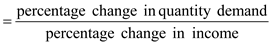# Quiz 5: Elasticity and Its Application

Business

Price elasticity of demand: If a life-saving medicine having no close substitute, then people will buy it irrespective of its price. Hence, the medicine will have small elasticity of demand. Hence, the option 'a' is correct.

Elasticity of demand is defined as the increase or decrease on the demand of a good due to the change in the price of the good. Demand is said to be elastic if demand changes a lot when the prices changes. (a) Required textbooks are a necessity and have no close substitutes. Thus, the demand for textbooks is inelastic. Mystery novels have close substitutes. Thus, their demand is elastic. Therefore, Mystery Novels have more elastic demand. (b) Beethoven recordings have more elastic demand than classical music recordings in general. Beethoven recordings are a narrower market than classical music recordings, so it is easy to find close substitutes for them. Therefore, Beethoven recordings have more elastic demand. (c) Subway rides during the next five years have more elastic demand than subway rides during the next six months. This is because goods tend to have more elastic demand over longer time horizons and inelastic demand over shorter time horizons. Therefore, Subway rides have more elastic demand. (d) Root beer has more elastic demand than water. Root beer is a luxury with close substitutes, while water is a necessity with no close substitutes. Therefore, root beer have more elastic demand.

Price Elasticity of Demand The price elasticity of demand measures how much the quantity demanded responds to a change in price. The price elasticity of demand for any good measures how willing consumers are to move away from the good as its price rises. Thus, the elasticity shows the many economic, social and psychological forces that shape consumer tastes. The price elasticity of demand is computed as follows. Price elasticity of demandDemand for a good is said to be elastic if the quantity demanded responds substantially to changes in the price. Demand is said to be inelastic if the quantity demanded responds only slightly to changes in the price. Income Elasticity of Demand The income elasticity of demand measures how the quantity demand changes as consumer income changes. It is calculated as the percentage change in the quantity demanded divided by the percentage change in income. That is, Income elasticity of demand